# Excel求和公式大全，多表、隔列、多条件求和

1、SUM求和快捷键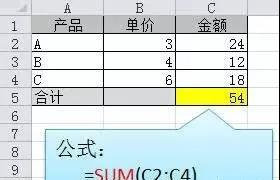2、巧设总计公式

=SUM(C2:C11)/2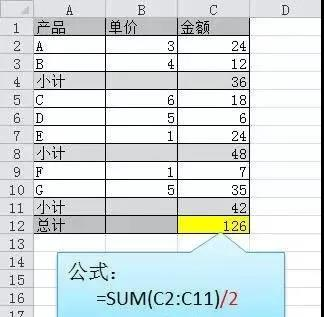3、隔列求和

=SUMIF(\$A\$2:\$G\$2,H\$2,A3:G3)

=SUMPRODUCT((MOD(COLUMN(B3:G3),2)=0)*B3:G3)

{=SUM(VLOOKUP(A3,A3:G3,ROW(1:3)*2,0))} 数组公式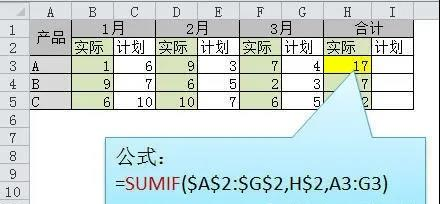4、单条件求和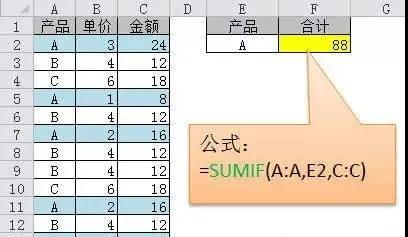5、单条件模糊求和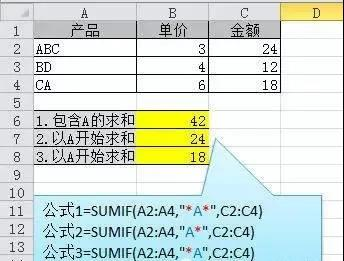6、多条件求和

=SUMIFS(数字区域,条件区域1，条件1，条件区域2，条件2....)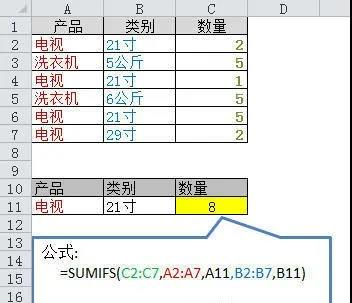7、多条件模糊求和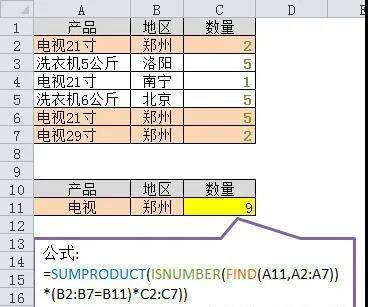=SUMIFS(C2:C7,A2:A7,A11&"*",B2:B7,B11)

-------------------------------------------

8、多表求和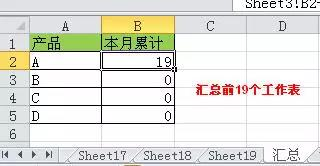=SUM(Sheet1:Sheet19!B2)

Sheet1:Sheet19 ：由第1工作表与最后一个工作表所组成的工作表集合。

=sum('*'!B2) ，在输入之后，公式会自动转为：=SUM(Sheet1:Sheet19!B2)

=SUM(Sheet1:AA!B2)

“AA”即是所添加的辅助工作表的名称。